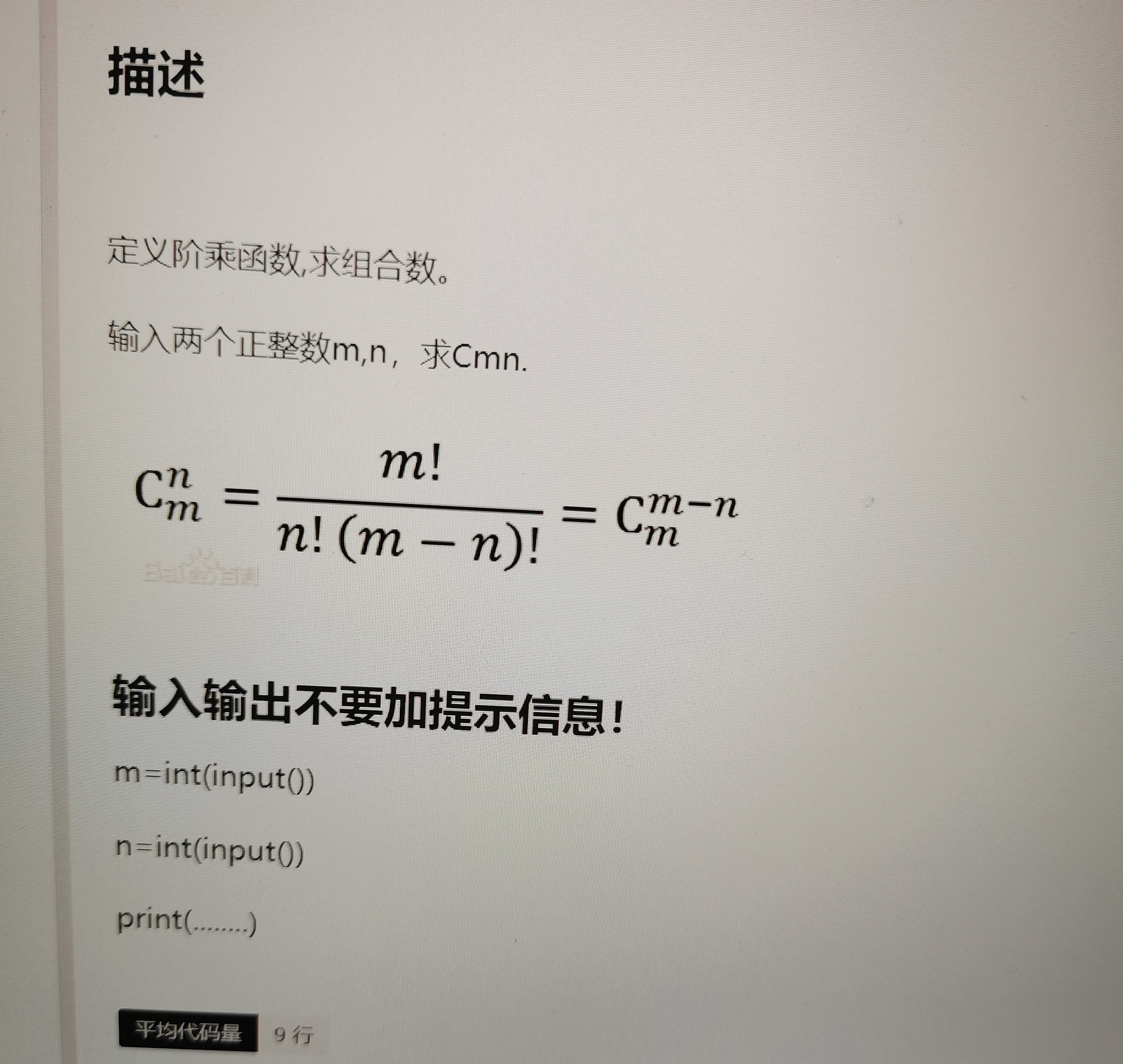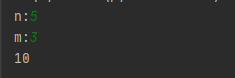2021-06-08 21:26

Python 定义阶乘函数，求组合数• 写回答
• 好问题 提建议
• 追加酬金
• 关注问题
• 收藏
• 邀请回答

2条回答默认 最新

•CSDN专家-黄老师 2021-06-08 21:29
已采纳
import math
n = int(input("n:"))
m = int(input("m:"))
print(math.factorial(n)//(math.factorial(m)*math.factorial(n-m)))如果对你有帮助，可以点击我这个回答右上方的【采纳】按钮，给我个采纳吗，谢谢

评论
解决 1 无用 1
打赏 举报